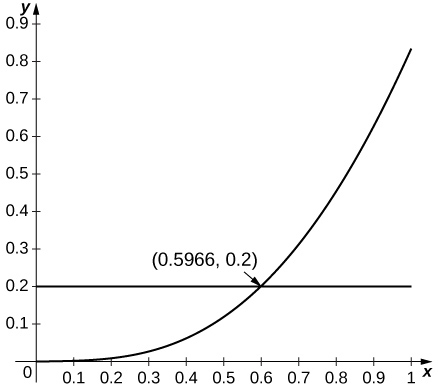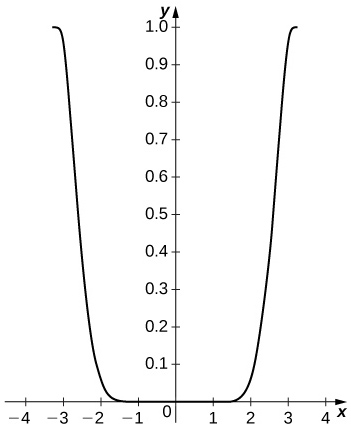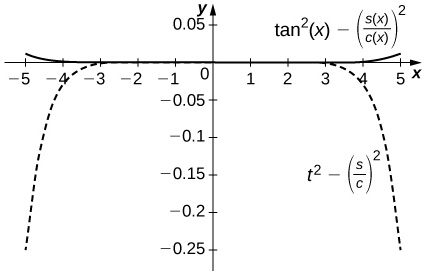$$\newcommand{\id}{\mathrm{id}}$$ $$\newcommand{\Span}{\mathrm{span}}$$ $$\newcommand{\kernel}{\mathrm{null}\,}$$ $$\newcommand{\range}{\mathrm{range}\,}$$ $$\newcommand{\RealPart}{\mathrm{Re}}$$ $$\newcommand{\ImaginaryPart}{\mathrm{Im}}$$ $$\newcommand{\Argument}{\mathrm{Arg}}$$ $$\newcommand{\norm}{\| #1 \|}$$ $$\newcommand{\inner}{\langle #1, #2 \rangle}$$ $$\newcommand{\Span}{\mathrm{span}}$$

# 10.3E: Exercises for Taylor Polynomials and Taylor Series

•• OpenStax
• OpenStax
$$\newcommand{\vecs}{\overset { \rightharpoonup} {\mathbf{#1}} }$$ $$\newcommand{\vecd}{\overset{-\!-\!\rightharpoonup}{\vphantom{a}\smash {#1}}}$$$$\newcommand{\id}{\mathrm{id}}$$ $$\newcommand{\Span}{\mathrm{span}}$$ $$\newcommand{\kernel}{\mathrm{null}\,}$$ $$\newcommand{\range}{\mathrm{range}\,}$$ $$\newcommand{\RealPart}{\mathrm{Re}}$$ $$\newcommand{\ImaginaryPart}{\mathrm{Im}}$$ $$\newcommand{\Argument}{\mathrm{Arg}}$$ $$\newcommand{\norm}{\| #1 \|}$$ $$\newcommand{\inner}{\langle #1, #2 \rangle}$$ $$\newcommand{\Span}{\mathrm{span}}$$ $$\newcommand{\id}{\mathrm{id}}$$ $$\newcommand{\Span}{\mathrm{span}}$$ $$\newcommand{\kernel}{\mathrm{null}\,}$$ $$\newcommand{\range}{\mathrm{range}\,}$$ $$\newcommand{\RealPart}{\mathrm{Re}}$$ $$\newcommand{\ImaginaryPart}{\mathrm{Im}}$$ $$\newcommand{\Argument}{\mathrm{Arg}}$$ $$\newcommand{\norm}{\| #1 \|}$$ $$\newcommand{\inner}{\langle #1, #2 \rangle}$$ $$\newcommand{\Span}{\mathrm{span}}$$

## Taylor Polynomials

In exercises 1 - 8, find the Taylor polynomials of degree two approximating the given function centered at the given point.

1) $$f(x)=1+x+x^2$$ at $$a=1$$

2) $$f(x)=1+x+x^2$$ at $$a=−1$$

$$f(−1)=1;\;f′(−1)=−1;\;f''(−1)=2;\quad p_2(x)=1−(x+1)+(x+1)^2$$

3) $$f(x)=\cos(2x)$$ at $$a=π$$

4) $$f(x)=\sin(2x)$$ at $$a=\frac{π}{2}$$

$$f′(x)=2\cos(2x);\;f''(x)=−4\sin(2x);\quad p_2(x)=−2(x−\frac{π}{2})$$

5) $$f(x)=\sqrt{x}$$ at $$a=4$$

6) $$f(x)=\ln x$$ at $$a=1$$

$$f′(x)=\dfrac{1}{x};\; f''(x)=−\dfrac{1}{x^2};\quad p_2(x)=0+(x−1)−\frac{1}{2}(x−1)^2$$

7) $$f(x)=\dfrac{1}{x}$$ at $$a=1$$

8) $$f(x)=e^x$$ at $$a=1$$

$$p_2(x)=e+e(x−1)+\dfrac{e}{2}(x−1)^2$$

## Taylor Remainder Theorem

In exercises 9 - 14, verify that the given choice of $$n$$ in the remainder estimate $$|R_n|≤\dfrac{M}{(n+1)!}(x−a)^{n+1}$$, where $$M$$ is the maximum value of $$∣f^{(n+1)}(z)∣$$ on the interval between $$a$$ and the indicated point, yields $$|R_n|≤\frac{1}{1000}$$. Find the value of the Taylor polynomial $$p_n$$ of $$f$$ at the indicated point.

9) [T] $$\sqrt{10};\; a=9,\; n=3$$

10) [T] $$(28)^{1/3};\; a=27,\; n=1$$

$$\dfrac{d^2}{dx^2}x^{1/3}=−\dfrac{2}{9x^{5/3}}≥−0.00092…$$ when $$x≥28$$ so the remainder estimate applies to the linear approximation $$x^{1/3}≈p_1(27)=3+\dfrac{x−27}{27}$$, which gives $$(28)^{1/3}≈3+\frac{1}{27}=3.\bar{037}$$, while $$(28)^{1/3}≈3.03658.$$

11) [T] $$\sin(6);\; a=2π,\; n=5$$

12) [T] $$e^2; \; a=0,\; n=9$$

Using the estimate $$\dfrac{2^{10}}{10!}<0.000283$$ we can use the Taylor expansion of order 9 to estimate $$e^x$$ at $$x=2$$. as $$e^2≈p_9(2)=1+2+\frac{2^2}{2}+\frac{2^3}{6}+⋯+\frac{2^9}{9!}=7.3887$$… whereas $$e^2≈7.3891.$$

13) [T] $$\cos(\frac{π}{5});\; a=0,\; n=4$$

14) [T] $$\ln(2);\; a=1,\; n=1000$$

Since $$\dfrac{d^n}{dx^n}(\ln x)=(−1)^{n−1}\dfrac{(n−1)!}{x^n},R_{1000}≈\frac{1}{1001}$$. One has $$\displaystyle p_{1000}(1)=\sum_{n=1}^{1000}\dfrac{(−1)^{n−1}}{n}≈0.6936$$ whereas $$\ln(2)≈0.6931⋯.$$

## Approximating Definite Integrals Using Taylor Series

15) Integrate the approximation $$\sin t≈t−\dfrac{t^3}{6}+\dfrac{t^5}{120}−\dfrac{t^7}{5040}$$ evaluated at $$π$$t to approximate $$\displaystyle ∫^1_0\frac{\sin πt}{πt}\,dt$$.

16) Integrate the approximation $$e^x≈1+x+\dfrac{x^2}{2}+⋯+\dfrac{x^6}{720}$$ evaluated at $$−x^2$$ to approximate $$\displaystyle ∫^1_0e^{−x^2}\,dx.$$

$$\displaystyle ∫^1_0\left(1−x^2+\frac{x^4}{2}−\frac{x^6}{6}+\frac{x^8}{24}−\frac{x^{10}}{120}+\frac{x^{12}}{720}\right)\,dx =1−\frac{1^3}{3}+\frac{1^5}{10}−\frac{1^7}{42}+\frac{1^9}{9⋅24}−\frac{1^{11}}{120⋅11}+\frac{1^{13}}{720⋅13}≈0.74683$$ whereas $$\displaystyle ∫^1_0e^{−x^2}dx≈0.74682.$$

## More Taylor Remainder Theorem Problems

In exercises 17 - 20, find the smallest value of $$n$$ such that the remainder estimate $$|R_n|≤\dfrac{M}{(n+1)!}(x−a)^{n+1}$$, where $$M$$ is the maximum value of $$∣f^{(n+1)}(z)∣$$ on the interval between $$a$$ and the indicated point, yields $$|R_n|≤\frac{1}{1000}$$ on the indicated interval.

17) $$f(x)=\sin x$$ on $$[−π,π],\; a=0$$

18) $$f(x)=\cos x$$ on $$[−\frac{π}{2},\frac{π}{2}],\; a=0$$

Since $$f^{(n+1)}(z)$$ is $$\sin z$$ or $$\cos z$$, we have $$M=1$$. Since $$|x−0|≤\frac{π}{2}$$, we seek the smallest $$n$$ such that $$\dfrac{π^{n+1}}{2^{n+1}(n+1)!}≤0.001$$. The smallest such value is $$n=7$$. The remainder estimate is $$R_7≤0.00092.$$

19) $$f(x)=e^{−2x}$$ on $$[−1,1],a=0$$

20) $$f(x)=e^{−x}$$ on $$[−3,3],a=0$$

Since $$f^{(n+1)}(z)=±e^{−z}$$ one has $$M=e^3$$. Since $$|x−0|≤3$$, one seeks the smallest $$n$$ such that $$\dfrac{3^{n+1}e^3}{(n+1)!}≤0.001$$. The smallest such value is $$n=14$$. The remainder estimate is $$R_{14}≤0.000220.$$

In exercises 21 - 24, the maximum of the right-hand side of the remainder estimate $$|R_1|≤\dfrac{max|f''(z)|}{2}R^2$$ on $$[a−R,a+R]$$ occurs at $$a$$ or $$a±R$$. Estimate the maximum value of $$R$$ such that $$\dfrac{max|f''(z)|}{2}R^2≤0.1$$ on $$[a−R,a+R]$$ by plotting this maximum as a function of $$R$$.

21) [T] $$e^x$$ approximated by $$1+x,\; a=0$$

22) [T] $$\sin x$$ approximated by $$x,\; a=0$$

Since $$\sin x$$ is increasing for small $$x$$ and since $$\frac{d^2}{dx^2}\left(\sin x\right)=−\sin x$$, the estimate applies whenever $$R^2\sin(R)≤0.2$$, which applies up to $$R=0.596.$$23) [T] $$\ln x$$ approximated by $$x−1,\; a=1$$

24) [T] $$\cos x$$ approximated by $$1,\; a=0$$

Since the second derivative of $$\cos x$$ is $$−\cos x$$ and since $$\cos x$$ is decreasing away from $$x=0$$, the estimate applies when $$R^2\cos R≤0.2$$ or $$R≤0.447$$.## Taylor Series

In exercises 25 - 35, find the Taylor series of the given function centered at the indicated point.

25) $$f(x) = x^4$$ at $$a=−1$$

26) $$f(x) = 1+x+x^2+x^3$$ at $$a=−1$$

$$(x+1)^3−2(x+1)^2+2(x+1)$$

27) $$f(x) = \sin x$$ at $$a=π$$

28) $$f(x) = \cos x$$ at $$a=2π$$

Values of derivatives are the same as for $$x=0$$ so $$\displaystyle \cos x=\sum_{n=0}^∞(−1)^n\frac{(x−2π)^{2n}}{(2n)!}$$

29) $$f(x) = \sin x$$ at $$x=\frac{π}{2}$$

30) $$f(x) = \cos x$$ at $$x=\frac{π}{2}$$

$$\cos(\frac{π}{2})=0,\;−\sin(\frac{π}{2})=−1$$ so $$\displaystyle \cos x=\sum_{n=0}^∞(−1)^{n+1}\frac{(x−\frac{π}{2})^{2n+1}}{(2n+1)!}$$, which is also $$−\cos(x−\frac{π}{2})$$.

31) $$f(x) = e^x$$ at $$a=−1$$

32) $$f(x) = e^x$$ at $$a=1$$

The derivatives are $$f^{(n)}(1)=e,$$ so $$\displaystyle e^x=e\sum_{n=0}^∞\frac{(x−1)^n}{n!}.$$

33) $$f(x) = \dfrac{1}{(x−1)^2}$$ at $$a=0$$ (Hint: Differentiate the Taylor Series for$$\dfrac{1}{1−x}$$.)

34) $$f(x) = \dfrac{1}{(x−1)^3}$$ at $$a=0$$

$$\displaystyle \frac{1}{(x−1)^3}=−\frac{1}{2}\frac{d^2}{dx^2}\left(\frac{1}{1−x}\right)=−\sum_{n=0}^∞\left(\frac{(n+2)(n+1)x^n}{2}\right)$$

35) $$\displaystyle F(x)=∫^x_0\cos(\sqrt{t})\,dt;\quad \text{where}\; f(t)=\sum_{n=0}^∞(−1)^n\frac{t^n}{(2n)!}$$ at a=0 (Note: $$f$$ is the Taylor series of $$\cos(\sqrt{t}).)$$

In exercises 36 - 44, compute the Taylor series of each function around $$x=1$$.

36) $$f(x)=2−x$$

$$2−x=1−(x−1)$$

37) $$f(x)=x^3$$

38) $$f(x)=(x−2)^2$$

$$((x−1)−1)^2=(x−1)^2−2(x−1)+1$$

39) $$f(x)=\ln x$$

40) $$f(x)=\dfrac{1}{x}$$

$$\displaystyle \frac{1}{1−(1−x)}=\sum_{n=0}^∞(−1)^n(x−1)^n$$

41) $$f(x)=\dfrac{1}{2x−x^2}$$

42) $$f(x)=\dfrac{x}{4x−2x^2−1}$$

$$\displaystyle x\sum_{n=0}^∞2^n(1−x)^{2n}=\sum_{n=0}^∞2^n(x−1)^{2n+1}+\sum_{n=0}^∞2^n(x−1)^{2n}$$

43) $$f(x)=e^{−x}$$

44) $$f(x)=e^{2x}$$

$$\displaystyle e^{2x}=e^{2(x−1)+2}=e^2\sum_{n=0}^∞\frac{2^n(x−1)^n}{n!}$$

## Maclaurin Series

[T] In exercises 45 - 48, identify the value of $$x$$ such that the given series $$\displaystyle \sum_{n=0}^∞a_n$$ is the value of the Maclaurin series of $$f(x)$$ at $$x$$. Approximate the value of $$f(x)$$ using $$\displaystyle S_{10}=\sum_{n=0}^{10}a_n$$.

45) $$\displaystyle \sum_{n=0}^∞\frac{1}{n!}$$

46) $$\displaystyle \sum_{n=0}^∞\frac{2^n}{n!}$$

$$x=e^2;\quad S_{10}=\dfrac{34,913}{4725}≈7.3889947$$

47) $$\displaystyle \sum_{n=0}^∞\frac{(−1)^n(2π)^{2n}}{(2n)!}$$

48) $$\displaystyle \sum_{n=0}^∞\frac{(−1)^n(2π)^{2n+1}}{(2n+1)!}$$

$$\sin(2π)=0;\quad S_{10}=8.27×10^{−5}$$

In exercises 49 - 52 use the functions $$S_5(x)=x−\dfrac{x^3}{6}+\dfrac{x^5}{120}$$ and $$C_4(x)=1−\dfrac{x^2}{2}+\dfrac{x^4}{24}$$ on $$[−π,π]$$.

49) [T] Plot $$\sin^2x−(S_5(x))^2$$ on $$[−π,π]$$. Compare the maximum difference with the square of the Taylor remainder estimate for $$\sin x.$$

50) [T] Plot $$\cos^2x−(C_4(x))^2$$ on $$[−π,π]$$. Compare the maximum difference with the square of the Taylor remainder estimate for $$\cos x$$.

The difference is small on the interior of the interval but approaches $$1$$ near the endpoints. The remainder estimate is $$|R_4|=\frac{π^5}{120}≈2.552.$$51) [T] Plot $$|2S_5(x)C_4(x)−\sin(2x)|$$ on $$[−π,π]$$.

52) [T] Compare $$\dfrac{S_5(x)}{C_4(x)}$$ on $$[−1,1]$$ to $$\tan x$$. Compare this with the Taylor remainder estimate for the approximation of $$\tan x$$ by $$x+\dfrac{x^3}{3}+\dfrac{2x^5}{15}$$.

The difference is on the order of $$10^{−4}$$ on $$[−1,1]$$ while the Taylor approximation error is around $$0.1$$ near $$±1$$. The top curve is a plot of $$\tan^2x−\left(\dfrac{S_5(x)}{C_4(x)}\right)^2$$ and the lower dashed plot shows $$t^2−\left(\dfrac{S_5}{C_4}\right)^2$$.53) [T] Plot $$e^x−e_4(x)$$ where $$e_4(x)=1+x+\dfrac{x^2}{2}+\dfrac{x^3}{6}+\dfrac{x^4}{24}$$ on $$[0,2]$$. Compare the maximum error with the Taylor remainder estimate.

54) (Taylor approximations and root finding.) Recall that Newton’s method $$x_{n+1}=x_n−\dfrac{f(x_n)}{f'(x_n)}$$ approximates solutions of $$f(x)=0$$ near the input $$x_0$$.

a. If $$f$$ and $$g$$ are inverse functions, explain why a solution of $$g(x)=a$$ is the value $$f(a)$$ of $$f$$.

b. Let $$p_N(x)$$ be the $$N^{\text{th}}$$ degree Maclaurin polynomial of $$e^x$$. Use Newton’s method to approximate solutions of $$p_N(x)−2=0$$ for $$N=4,5,6.$$

c. Explain why the approximate roots of $$p_N(x)−2=0$$ are approximate values of $$\ln(2).$$

b. The following are the $$x_n$$ values after $$10$$ iterations of Newton’s method to approximation a root of $$p_N(x)−2=0$$: for $$N=4,x=0.6939...;$$ for $$N=5,x=0.6932...;$$ for $$N=6,x=0.69315...;.$$ (Note: $$\ln(2)=0.69314...$$)

## Evaluating Limits using Taylor Series

In exercises 55 - 58, use the fact that if $$\displaystyle q(x)=\sum_{n=1}^∞a_n(x−c)^n$$ converges in an interval containing $$c$$, then $$\displaystyle \lim_{x→c}q(x)=a_0$$ to evaluate each limit using Taylor series.

55) $$\displaystyle \lim_{x→0}\frac{\cos x−1}{x^2}$$

56) $$\displaystyle \lim_{x→0}\frac{\ln(1−x^2)}{x^2}$$

$$\dfrac{\ln(1−x^2)}{x^2}→−1$$

57) $$\displaystyle \lim_{x→0}\frac{e^{x^2}−x^2−1}{x^4}$$

58) $$\displaystyle \lim_{x→0^+}\frac{\cos(\sqrt{x})−1}{2x}$$

$$\displaystyle \frac{\cos(\sqrt{x})−1}{2x}≈\frac{(1−\frac{x}{2}+\frac{x^2}{4!}−⋯)−1}{2x}→−\frac{1}{4}$$